Function Repository Resource:

# NormalSurface

Compute the normal surface of a curve

Contributed by: Alfred Gray
 ResourceFunction["NormalSurface"][c,t,{u,v}] gives the normal surface of a curve c with parameter t using u and v to parametrize the result.

## Details and Options

The normal surface associated to a curve is generated by its normal vector field.
The normal surface is effectively a ruled surface.

## Examples

### Basic Examples (4)

Define and plot a Viviani’s curve:

 In:=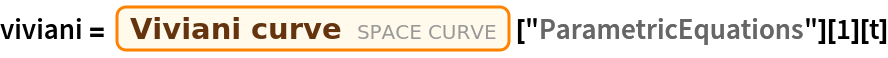Out=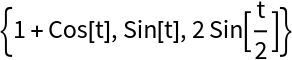In:=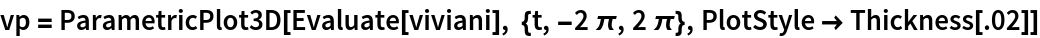Out=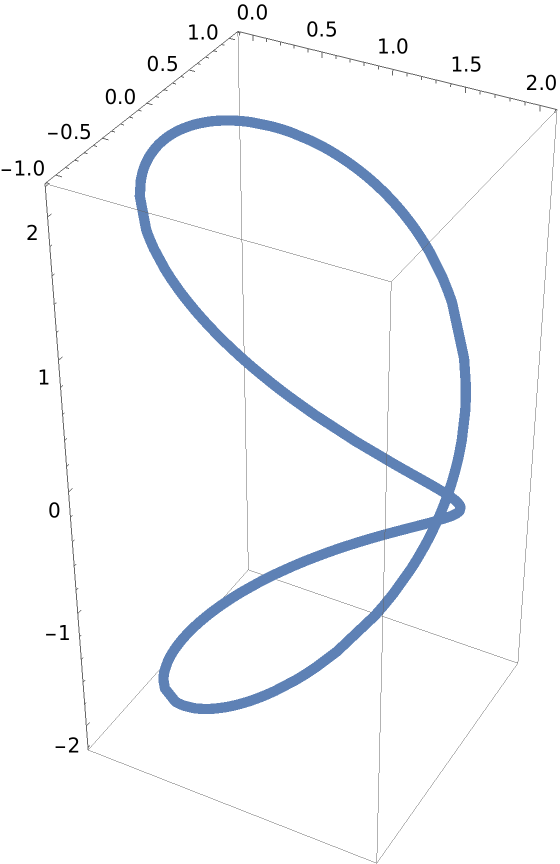Compute the normal surface of Viviani’s curve:

 In:=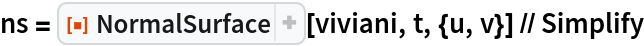Out=In:=Out=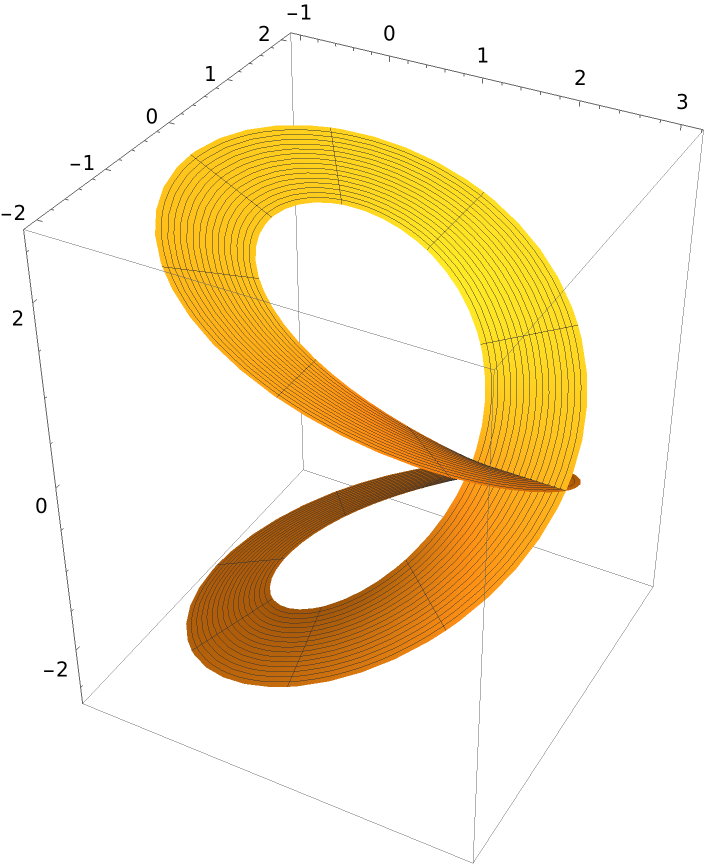Compute the binormal surface of Viviani’s curve with the resource function BiormalSurface and plot the result:

 In:=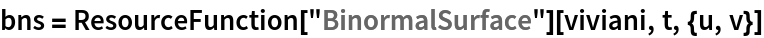Out=In:=Out=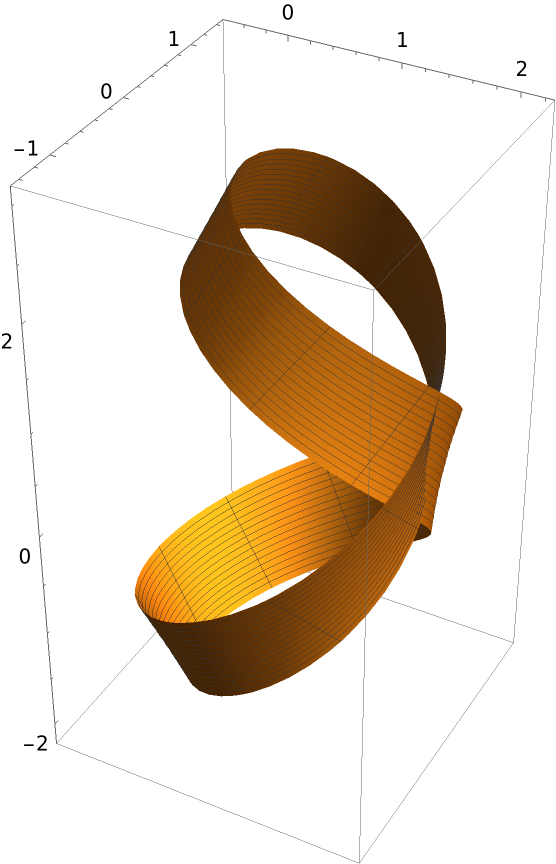Show both surfaces along with Viviani’s curve:

 In:=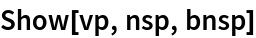Out=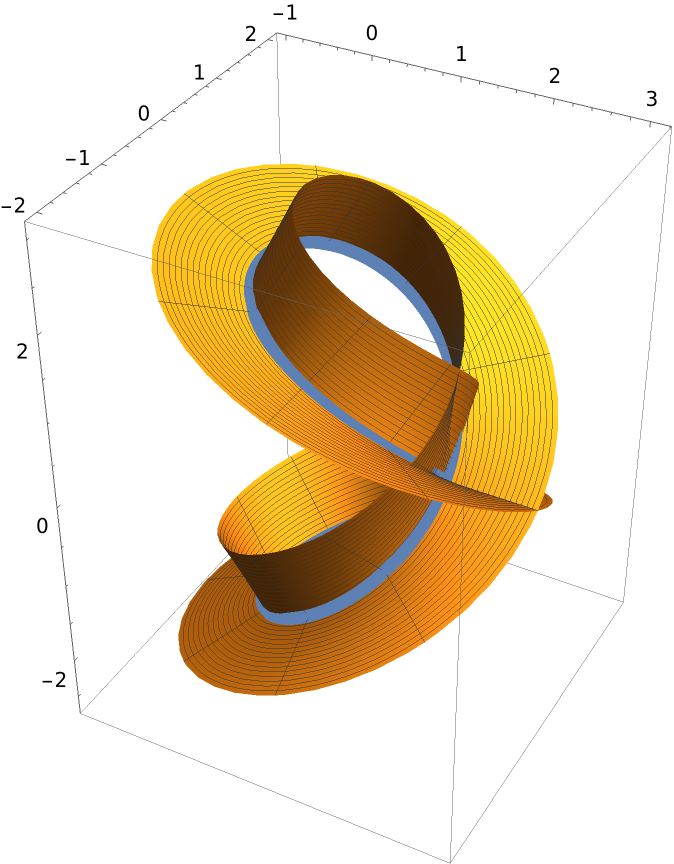Enrique Zeleny

## Version History

• 1.0.0 – 24 February 2020